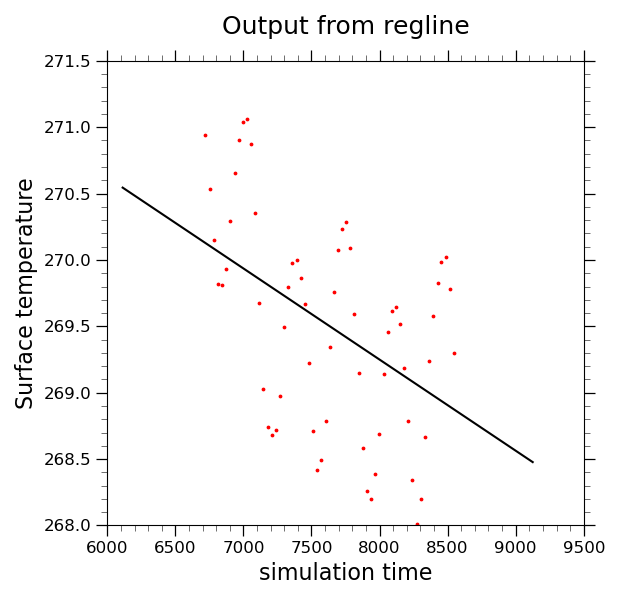# NCL_scatter_4.py#

This script illustrates the following concepts:
• Drawing a scatter plot with a regression line

• Drawing a time series plot

• Calculating the least squared regression for a one dimensional array

• Smoothing data so that seasonal cycle is less prominent

• Changing the markers in an XY plot

• Changing the marker color in an XY plot

• Changing the marker size in an XY plot

See following URLs to see the reproduced NCL plot & script:

Import packages:

```import numpy as np
import xarray as xr
import matplotlib.pyplot as plt

import geocat.datafiles as gdf
import geocat.viz as gv
```

```# Open a netCDF data file using xarray default engine and load the data into xarrays
ds = xr.open_dataset(gdf.get("netcdf_files/b003_TS_200-299.nc"),
decode_times=False)
# Extract variable
ts = ds.TS.sel(lat=60, lon=180, method='nearest')
```

Preprocess data:

```# Smooth data so that seasonal cycle is less prominent.
# This is for demo purposes only  so that the regression line is more sloped.
ts_rolled = ts.rolling(time=40, center=True).mean().dropna('time')

# Calculate regression line
m, b = np.polyfit(ts_rolled.time, ts_rolled.values, 1)
regline_vals = [m * x + b for x in ts.time]
```

Plot:

```# Generate figure (set its size (width, height) in inches) and axes
plt.figure(figsize=(6.2, 6))
ax = plt.gca()

# Scatter-plot the data
plt.scatter(ts_rolled.time, ts_rolled.values, c='red', s=3)

# Plot a regression line
plt.plot(ts.time, regline_vals, 'black')

# specify X and Y axis limits
plt.xlim([6000, 9500])
plt.ylim([268.0, 271.5])

# Use geocat.viz.util convenience function to add minor and major tick lines
x_minor_per_major=5,
y_minor_per_major=5,
labelsize=12)

# Use geocat.viz.util convenience function to set titles and labels without calling several matplotlib functions
gv.set_titles_and_labels(ax,
maintitle="Output from regline",
xlabel="simulation time",
ylabel="Surface temperature")

# Show the plot
plt.tight_layout()
plt.show()
```Total running time of the script: ( 0 minutes 0.352 seconds)

Gallery generated by Sphinx-Gallery